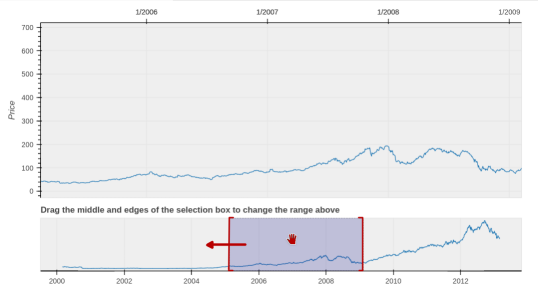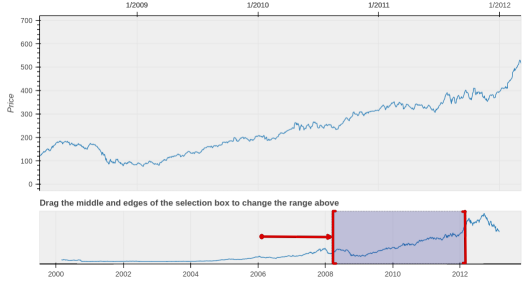# Getting selected elements by a time series range. BokehAs a newcomer to Bokeh, i would like to know what the events have to be handled to figure out the selected elements on a timeseris plot by a range tool to process them further on a server side and what an object receives those events, Plot or RangeTool.

In the case of using a range tool, elements aren't selected but the start and end of the range dates are, therefore, a final array of them is extracted manually from a data source.

The Bokeh documentation doesn't answer the questions, so a straight way to get to know it is examining all the events of both objects.

## Prepare a test stand

First of all, the test stand is run as a server to run python callbacks for handling events. The example is taken as a basis to extend.

stand.py

``````from functools import partial

import numpy as np
import bokeh.events
from bokeh.models import ColumnDataSource, RangeTool
from bokeh.plotting import figure, show
from bokeh.sampledata.stocks import AAPL
from bokeh.layouts import column
from bokeh.application.handlers.function import FunctionHandler
from bokeh.application.application import Application
from bokeh.server.server import Server

def make_models():
dates = np.array(AAPL['date'], dtype=np.datetime64)

data_plot = figure(height=300, width=800, tools="xpan", toolbar_location=None,
x_axis_type="datetime", x_axis_location="above",
background_fill_color="#efefef", x_range=(dates, dates))

data_plot.line('date', 'close', source=source)
data_plot.yaxis.axis_label = 'Price'

range_plot = figure(title="Drag the middle and edges of the selection box to change the range above",
height=130, width=800, y_range=data_plot.y_range,
x_axis_type="datetime", y_axis_type=None,
tools="", toolbar_location=None, background_fill_color="#efefef")

range_tool = RangeTool(x_range=data_plot.x_range)
range_tool.overlay.fill_color = "navy"
range_tool.overlay.fill_alpha = 0.2

range_plot.line('date', 'close', source=source)
range_plot.ygrid.grid_line_color = None
range_plot.toolbar.active_multi = range_tool

return data_plot, range_plot, range_tool

def make_layout():
data_plot, range_plot, range_tool = make_models()
layout = column(data_plot, range_plot)
return layout

def make_doc(doc):
l = make_layout()
return doc

app = Application(FunctionHandler(make_doc))
srv.run_until_shutdown()
``````

## Print out events data

Objects `range_plot, range_tool` are the targets to examine. Here is a function to assign a callback to the each event of both of them both. A callback prints event data as is to guess what the specific types containing a selected range we are looking for.

### Plot events

Plot events are assigned by the `on_event` method, it tracks many UI element interations in comparison to `on_change`, which tracks the object attributes changes.

During running, a few of the events riase an execption an have to be excluded from a callback list:

``````AttributeError: type object 'DocumentEvent' has no attribute 'event_name'
``````

Define a callback and assing it to:

``````def assign_callbacks(plot):

def _print_out_callback(event_name, *args):
print('Event: ', event_name, 'Data: ', args)

event_names = list(bokeh.events.__all__)
for excluded in ('DocumentEvent', 'Event', 'ModelEvent', 'PlotEvent', 'PointEvent'):
event_names.remove(excluded)

for event_name in event_names:
event = getattr(bokeh.events, event_name)
plot.on_event(event, partial(_print_out_callback, event_name))

def make_layout():
data_plot, range_plot, range_tool = make_models()

assign_callbacks(range_plot)
layout = column(data_plot, range_plot)
return layout
``````

All the time the following events are emitted in plenty and should be ignored, we often move a mouse cursor:

``````Event:  MouseEnter Data:  (<bokeh.events.MouseEnter object at 0x7fc53a492a60>,)
Event:  MouseMove Data:  (<bokeh.events.MouseMove object at 0x7fc53a492c70>,)
Event:  MouseMove Data:  (<bokeh.events.MouseMove object at 0x7fc53a492400>,)
Event:  MouseMove Data:  (<bokeh.events.MouseMove object at 0x7fc53a492b20>,)
``````

While moving a UI selection element to the left on the `range_plot` the follow events happen:``````Event:  Press Data:  (<bokeh.events.Press object at 0x7fc53a492970>,)
Event:  PanStart Data:  (<bokeh.events.PanStart object at 0x7fc53a492730>,)
Event:  Pan Data:  (<bokeh.events.Pan object at 0x7fc53a492c10>,)
...
Event:  Pan Data:  (<bokeh.events.Pan object at 0x7fc53a4927f0>,)
Event:  RangesUpdate Data:  (<bokeh.events.RangesUpdate object at 0x7fc53a492400>,)
Event:  PanEnd Data:  (<bokeh.events.PanEnd object at 0x7fc53a492d90>,)
``````

Ok, the output is bringing us to the idea that the events `Press, Pan, PanStart, PanEnd, RangesUpdate` can contain the targets. This list i would reduce to three of them `PanStart, PanEnd, RangesUpdate` due to their name semantic fits more to obtaining the range's start and end dates. As specfific events are found, the callback can be extended by printing their attributes:

``````def _print_out_callback(event_name, *args):
if event_name in {'PanStart', 'PanEnd'}:
event = args
print('Event: ', event_name, f'Data: {event.sx}, {event.sy}, {event.x}, {event.y}')
elif event_name == 'RangesUpdate':
event = args
print('Event: ', event_name, f'Data: {event.x0}, {event.y0}, {event.x1}, {event.y1}')
else:
print('Event: ', event_name, 'Data: ', args)
``````

And output looks now:

``````Event:  PanStart Data: x=1199109699452.7036, sx=494.0841155052185, y=339.97113540536384, sy=68.16104125976562
Event:  RangesUpdate Data: x0=931357440000, x1=1382607360000, y0=-27.589000000000055, y1=719.729
Event:  PanEnd Data: x=1259761071764.9924, sx=593.545663356781, y=339.97113540536384, sy=68.16104125976562
``````

`PanStart.x, PanEnd.x` timestamps look accurate in comparison to `RangeUpdate.x0, RangeUpdate.x1`.

### Tool events

`range_tool` object is not a subclass of the `Plot` model, but `Tool` subclass, so `on_change` handler is assigned to it. Targets now are two object attributes `range_tool.x_range.start, range_tool.x_range.end` on `x_range` object of class Range1d .

``````def on_change_callback(attr, old, new):
print(f'Attr: {attr}, old={old}, new={new}')

def make_layout():
data_plot, range_plot, range_tool = make_models()

# assign_callbacks(range_plot)
print('Initital values.', 'start=', range_tool.x_range.start, 'end=', range_tool.x_range.end)
range_tool.x_range.on_change('start', on_change_callback)
range_tool.x_range.on_change('end', on_change_callback)
layout = column(data_plot, range_plot)
return layout
``````
``````Initital values. start= 2006-02-17 end= 2010-02-09
Attr: start, old=2006-02-17, new=1146842169081.081
Attr: end, old=2010-02-09, new=1272381369081.081
...
Attr: start, old=1204772902054.0552, new=1205382699243.2444
Attr: end, old=1330312102054.054, new=1330921899243.2432
``````

Check the timestamps whether they match the selection range position on the picture.``````In : from datetime import datetime

In : datetime.utcfromtimestamp(1205382699243/1000)
Out: datetime.datetime(2008, 3, 13, 4, 31, 39, 243000)

In : datetime.utcfromtimestamp(1330921899243/1000)
Out: datetime.datetime(2012, 3, 5, 4, 31, 39, 243000)
``````

### Final selection computation

Summing up the results of the above exploration, a final selection is easily found by handling the event `PanEnd` and converting timestamp attributes of the `range_tool.x_range` object to datetime.

``````from datetime import datetime

def pan_end_callback(selected_range, event):
start= datetime.utcfromtimestamp(selected_range.start/1000)
end = datetime.utcfromtimestamp(selected_range.end/1000)
print(f'Attrs on PanEnd: start={start}, end={end}')

def make_layout():
data_plot, range_plot, range_tool = make_models()

range_plot.on_event(bokeh.events.PanEnd, partial(pan_end_callback, range_tool.x_range))
layout = column(data_plot, range_plot)
return layout
``````

Sample output:

``````Attrs on PanEnd: start=2007-09-11 16:26:12.324324, end=2011-09-03 16:26:12.324324
``````

## Selected elemets

The complete stand.py test stand script.

With the values returned by the `pan_end_callback` the data source `source` can be filtered out to extract elements for further server side processing.

``````In : date_val = zip(source.data['date'], source.data['close'])
In : date_val_sorted = sorted(date_val, key=lambda pair: pair)
In : date_val_filtered = filter(lambda pair: datetime(2008, 3, 13, 4, 31, 39, 243000).date()
<= pair <=
datetime(2012, 3, 5, 4, 31, 39, 243000).date(),
date_val_sorted)
``````